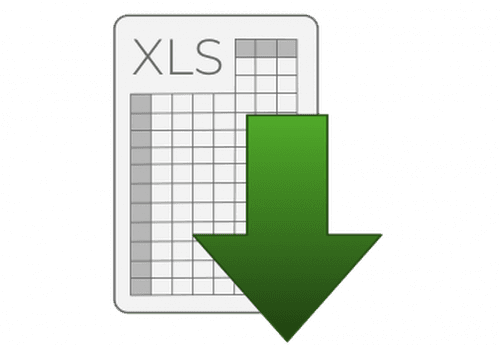Excel - A macro to perform the automatic numbering of invoices

In this article we will show you an easy way of doing an automatic numbering in Excel using a MACRO.How to do it

For example, this MACRO can increment an invoice number. Here, the number is displayed in cell "A1", but it can be changed to your convenience:

Sub Increment_invoice()
Dim num As Integer
Range("A1").Select
num = Range("A1").Value
num = num + 1
Range("A1").Value = num
End Sub

You should also add these lines to the end of a macro so that every time you print your invoice, the next one will be incremented automatically as follows:

Sub PRINT()
ActiveWindow.SelectedSheets.PrintOut Copies:=1, Collate:=True
Dim num As Integer
Range("A1").Select
num = Range("A1").Value
num = num + 1
Range("A1").Value = num
End Sub